# Maths Applications for Android

Solve all kinds of complex math calculations for any kind of project or job thanks to these maths applications for Android smartphones and tablets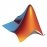Use MATLAB remotely on Android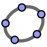GeoGebra 5.0.761.0

The Android version of the mathematical operations program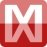Mathway 5.2.0

Application to solve math problems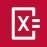Photomath 8.20.0

Solve any maths operation with your Android's camera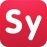Symbolab 10.1.1

Solve those impossible math problems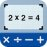Solve mathematical operations with a photo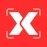Gauthmath 1.5.9

The app that solves all mathematical problems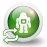Unit Converter 2.8.5.22

Convert any measurement and monetary unit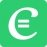Cymath 2.44

Solve mathematical operations in an instant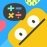Todo Math 6.13.2

Fun math for beginners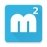MalMath 6.0.18

Solve mathematical operations step by step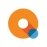Qanda 5.2.22

Solve math problems with the help of the camera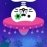A complete early-learning mathematics course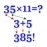Have fun with math games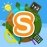Smartick 1.8.1

Learn math while playing with this educational app